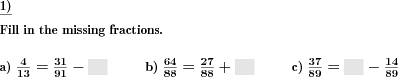Custom math worksheets at your fingertips# Details for problem "Fractions addition subtraction fill in the blanks"

Quickname: 4288

Elementary School, Primary School, Junior High School, Middle School, High School.

## Summary

In a sum term of fractions, blanks have to be filled in correctly

## Example## Description

Addition or subtraction terms with two fractions are given, as is the result. One of the fractions has been replaced by a blank space. The correct fraction has to be filled in.

The number of problems is selectable. The numerator and denominator of each fraction is taken from a preset number range.

Fractions may be unlike quantities, in which case they have to be converted to like quantities first. The problem may be configured to produce only series of like quantities - or only unlike quantities.

Improper fractions can be allowed, disallowed or enforced. In the case that they may occur, it can be chosen whether they will be output as improper fractions or mixed numbers.

Download free printable worksheets for this math problem here. The worksheet contains the problems only, the solution sheet includes the answers. Just click on the respective link.

•Worksheet 1Solution sheet with answers
•Worksheet 2Solution sheet with answers
•Worksheet 3Solution sheet with answers

If you can not see the solution sheets for download, they may be filtered out by an ad blocker that you may have installed. If this is the case, please allow ads for this page and reload the page. The solution sheets will then reappear.

• Do these sample worksheets do not really fit?
• Do you need more math worksheets, with a different level of difficulty?
• Would you like to combine different problems on a worksheet and adjust them to your needs?
• As a teacher, you can put together your own worksheets using the automatically generated math problems provided.
With a free initial credit, you can start creating your own math worksheets in a few minutes.

You can try it for free! Register here, to create custom worksheets now!

## Customization options for this problem

Parameter
Possible values
Number of problems
1, 2, 3, 4, 5, 6, 7, 8, 9, 10
Plus/Minus
Mixed, Plus, Minus
Number range
20, 30, 40, 50, 100, 200, 500, 1000, 10000, 100000
Improper fractions
No, Yes, as a fraction, Yes, as a mixed number
All like quantities
Never, May, Always

## Similar problems

Remark
Description
Plain addition / subtraction problem with result to be calculated